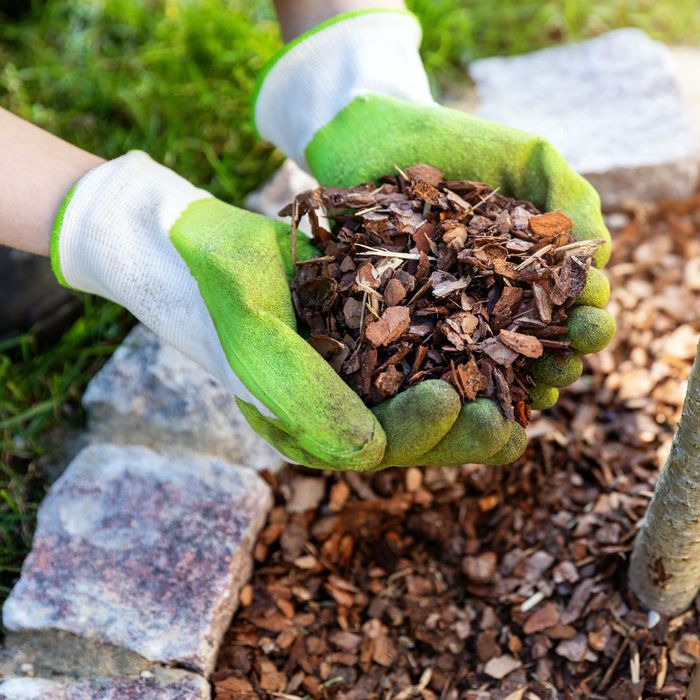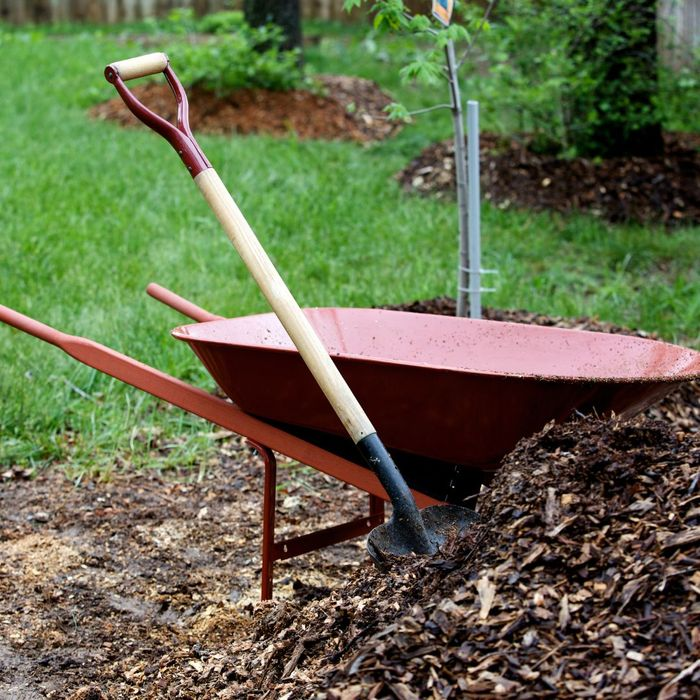## How to Estimate the Amount of Mulch Needed for Your Landscaping## Measure the Area

The first step to estimating the amount of mulch you need is to measure the area. Measure the length and width of the area, then multiply those two numbers together to get the total square footage. This will be the basis for your mulch calculations.## Calculate by Depth

Once you’ve determined the square footage of the area, you’ll need to decide how deep you want your mulch to be. Generally, a depth of two to three inches is recommended. Once you’ve determined the depth, you’ll need to multiply that number by the square footage you calculated earlier. This will give you the total number of cubic feet of mulch you’ll need for your project.## Consider the Type of Mulch You Are Using

Different types of mulch have different densities and will require different amounts of coverage. For example, a cubic yard of bark mulch will cover an area of up to 162 square feet, while a cubic yard of pine straw will cover up to 108 square feet.